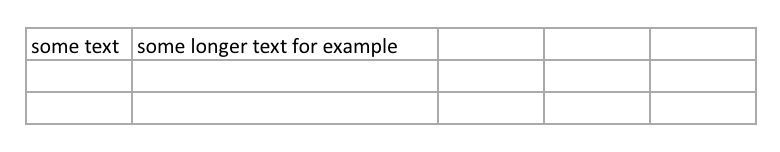# Ultra Fast Excel Writer for Ruby

``````require 'fast_excel'

workbook = FastExcel.open("hello_world.xlsx", constant_memory: true)
workbook.default_format.set(
font_size: 0, # user's default
font_family: "Arial"
)

bold = workbook.bold_format
worksheet.set_column(0, 0, FastExcel::DEF_COL_WIDTH, bold)

price = workbook.number_format("#,##0.00")
worksheet.set_column(1, 1, 20, price)

date_format = workbook.number_format("[\$-409]m/d/yy h:mm AM/PM;@")
worksheet.set_column(2, 2, 20, date_format)

worksheet.append_row(["message", "price", "date"], bold)

for i in 1..1000
worksheet.append_row(["Hello", (rand * 10_000_000).round(2), Time.now])
end

worksheet.append_row(["Sum", FastExcel::Formula.new("SUM(B2:B1001)")], bold)

workbook.close
``````

This repository and gem contain sources of libxlsxwriter

## Install

``````# Gemfile
gem 'fast_excel'
``````

Or

``````gem install fast_excel
``````

### Create Document

``````workbook = FastExcel.open # creates tmp file
# ...
``````

Also can use `workbook.remove_tmp_folder` to delete tmp file manually

Constant memory mode: saves each row to disk, good for really big files but can not change previous lines that already saved

``````workbook = FastExcel.open(constant_memory: true)
``````

Save to file

``````workbook = FastExcel.open("my_dinner.xlsx")
``````

### Write Data

FastExcel will automatically detect data type and one of `write_number` or `write_datetime` or `write_formula` or `write_string` or `write_url`

``````workbook = FastExcel.open

# write specific type value value
worksheet.write_number(row = 0, col = 5, 1_234_567, format = nil)

# write value with type detection
worksheet.write_value(row = 0, col = 5, 1_234_567, format = nil)

# write row of values. format argument can be format object or array of format objects
worksheet.write_row(row = 1, ["strong", 123_456, Time.now], format = nil)

# write row to the bottom
worksheet.append_row(["strong", 123_456, Time.now], )

# shortcut for append_row()
worksheet << ["strong", 123_456, Time.now]
``````

Saving dates: excel store dates as number of days since 1st January 1900, and FastExcel will make it for you.

To make saving of dates slightly faster can use `FastExcel.date_num` helper:

``````date_format = workbook.number_format("[\$-409]m/d/yy hh:mm;@")
worksheet.write_number(0, 0, FastExcel.date_num(Time.now, Time.zone.utc_offset), date_format)
``````

Formulas: special type of value in excel

``````worksheet << [1, 2, 3, 4]
worksheet << [FastExcel::Formula.new("SUM(A1:D1)")] # A2 will be shown as 10
``````

URL: Link to website or something else

``````url_format = workbook.add_format(underline: :underline_single, font_color: :blue) # format is optional
worksheet.append_row([
FastExcel::URL.new("https://github.com/Paxa/fast_excel"),
FastExcel::URL.new("postgres://localhost")
], url_format)
# or
worksheet.write_url(0, 2, "https://github.com/Paxa/fast_excel", url_format)
``````

### Data Formatting

``````format = workbook.add_format(
bold: true,
italic: true,
font_outline: true,
text_wrap: true,
font_strikeout: true,
shrink: true,
text_justlast: true,
font_size: 13, # default is 11, use 0 for user's default
font_name: "Arial", # default is Calibri, also accessible via font_family
font_color: :orange, # can use RGB hex as "#FF0000" or 0x00FF00 or color name as symbol or string
font_script: :font_subscript,
rotation: 10,
underline: :underline_single, # or :underline_double or :underline_single_accounting or :underline_double_accounting
indent: 1,
# border styles
border: :border_thin,
left: :medium,
top: :dashed,
right: :double,
bottom: :hair,
bottom_color: :alice_blue,
top_color: "#11ABCD",
# Align
align: {h: :align_center, v: :align_vertical_center},
num_format: "#,##0.00"
)
``````

Shortcuts:

``````workbook.bold_format # bold text
workbook.number_format("[\$-409]m/d/yy h:mm AM/PM;@") # format for date
``````

### Set Column Width

``````worksheet.set_column(start_col, end_col, width = nil, format = nil)
# or
worksheet.set_column_width(col, width = 60)
# or
worksheet.set_columns_width(start_col, end_col, width = 60)
``````

### Set Row Height

``````worksheet.set_row(row_num = 0, height = 30, format = nil)
``````

### Column Auto Width

Column authwidth only works for string values, because numbers may have custom formatting

Enabling column auto widths will slow down writing string values for about 15-25%

``````require 'fast_excel'

workbook = FastExcel.open(constant_memory: true)

worksheet.auto_width = true

worksheet.append_row(["some text", "some longer text for example"])

File.open('./some_file.xlsx', 'wb') {|f| f.write(content) }
``````### API

This gem is FFI binding for libxlsxwriter C library with some syntax sugar. All original functions is avaliable, for example:

``````Libxlsxwriter.worksheet_activate(worksheet) # => will call void worksheet_activate(lxw_worksheet *worksheet)
# or shorter:
worksheet.activate
``````

Full libxlsxwriter documentation: http://libxlsxwriter.github.io/

Generated rdoc: rubydoc.info/github/Paxa/fast_excel

## Benchmarks

1000 rows:

``````Comparison:
FastExcel:       31.7 i/s
Axlsx:        8.0 i/s - 3.98x  slower
write_xlsx:        6.9 i/s - 4.62x  slower
``````

20000 rows:

``````Comparison:
FastExcel:        1.4 i/s
Axlsx:        0.4 i/s - 3.46x  slower
write_xlsx:        0.1 i/s - 17.04x  slower
``````

Max memory usage, generating 100k rows:

``````FastExcel   - 20 MB
Axlsx       - 60 MB
write_xlsx - 100 MB
``````